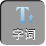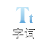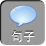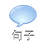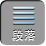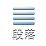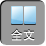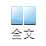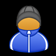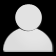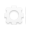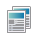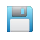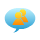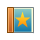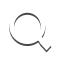-AA+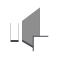⑴经过整理后是一个整式方程；

⑵只含有一个未知数；

⑶未知数的最高次数是 2.

1、判断下列方程，哪些是一元二次方程？

x 3x 25= 0；②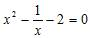；③ x 2= 1；④ ax 2bxc= 0；⑤( a 21) x 2ax5= 0；⑥ x 22 xyy 2= 1；⑦ x( x1)= x 22；⑧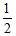x 2= x.

2、请写出一元二次方程( 12 x)( x4)= 2 x 23中的二次项、一次项、常数项及二次项系数和一次项系数.

3、若关于 x的方程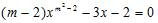是一元二次方程，求 m的值.

1.下列方程中，为一元二次方程的是( )

A. 3( x1) 2= 2( x1) B.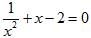C. ax 2bxc= 0 D. x 22 x=( x1)( x1)

2.若关于 x的方程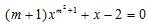是一元二次方程，则 m=.

3.指出一元二次方程 2 x 23 x= 1x( x3)中的二次项、一次项系数和常数项.

1. A

2. m= 1

3.二次项是 3 x 2、一次项系数是 0，常数项是－ 1.